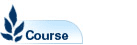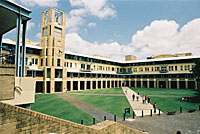# Computational Mathematics - MATH3101

Faculty: Faculty of Science

Course Outline: http://www.maths.unsw.edu.au/

Campus: Sydney

Units of Credit: 6

Indicative Contact Hours per Week: 4

Enrolment Requirements:

Prerequisite: 12 units of credit in Level 2 Math courses including (MATH2011 or MATH2111 and MATH2120 or MATH2130 or MATH2121 or MATH2221), or (both MATH2019 (DN) and MATH2089), or (both MATH2069 (CR) and MATH2099)

Excluded: MATH5305

Tuition Fee: See Tuition Fee Schedule

Further Information: See Class Timetable

View course information for previous years.

## Description

Partial differential equations provide the natural mathematical description of many phenomena of
interest in science, engineering and finance. Such equations are often difficult or impossible to solve using purely analytical (pencil and paper) methods, especially for realistic industrial problems. This course introduces finite difference methods for three key classes of partial differential equations: elliptic (Poisson equation), parabolic (heat or diffusion equation) and hyperbolic (transport equation). Related methods in computational linear algebra are also discussed.

The course includes a substantial practical component dealing with the computer implementation of the algorithms used for solving partial differential equations, as well as a broader discussion of scientific software development.

Note:Students must have some prior experience with simple computer programming.